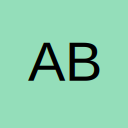# Insert formula into a range of cells

• I am trying to use a simple code to insert a formula into a range of cells, but I keep getting a Run-time error 1004. Can someone explain what I am doing wrong?

Code
``````Sub FormulaTest()
'
' FormulaTest Macro
'
'
Range("B2:B7").Formula = "=IFERROR(INDEX(AllParts.xlsx!\$B:\$B,MATCH(\$A3,AllParts!\$A:\$A,0)),"")"
End Sub``````
• Re: Insert formula into a range of cells

In VBA you must double-up the quotes

Code
``````Sub FormulaTest()
'
' FormulaTest Macro
'
'
Range("B2:B7").Formula = "=IFERROR(INDEX(AllParts.xlsx!\$B:\$B,MATCH(\$A3,AllParts!\$A:\$A,0)),"""")"
End Sub``````
• Re: Insert formula into a range of cells

Awesome, it worked. Thank you very much

## Participate now!

Don’t have an account yet? Register yourself now and be a part of our community!